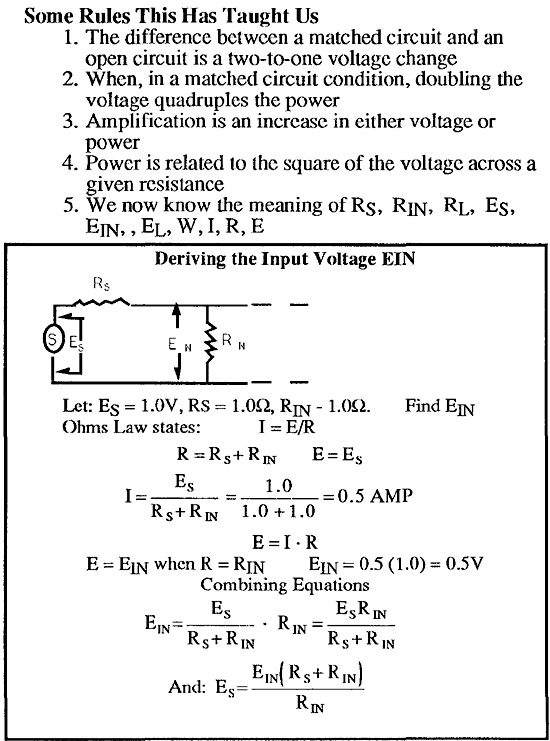# W.I.R.E. from SAC Newsletter Spring 1993

## Ohms law is expressed as: E = IR and W = EI

The potential force (E) is the product of the current (I) and the resistance (R) and: The power (W) is the product of the voltage (E) and the current (I).

From these two simple equations the WIRE wheel is generated. See Figure 1.

### Audio Systems

In audio systems, the primary use we make of Ohms law is to find power and voltage values. Current is rarely measured. Typically we deal with either a source or a load.

### Available Power

A source is said to develop its maximum available power when the load presented to it equals its source resistance.

See Figure 2.

This would mean that the black box was an amplifier because the power went up from 0.25 W to 1.0 W (a 4 to 1 ratio). Our voltage, however, merely doubled.